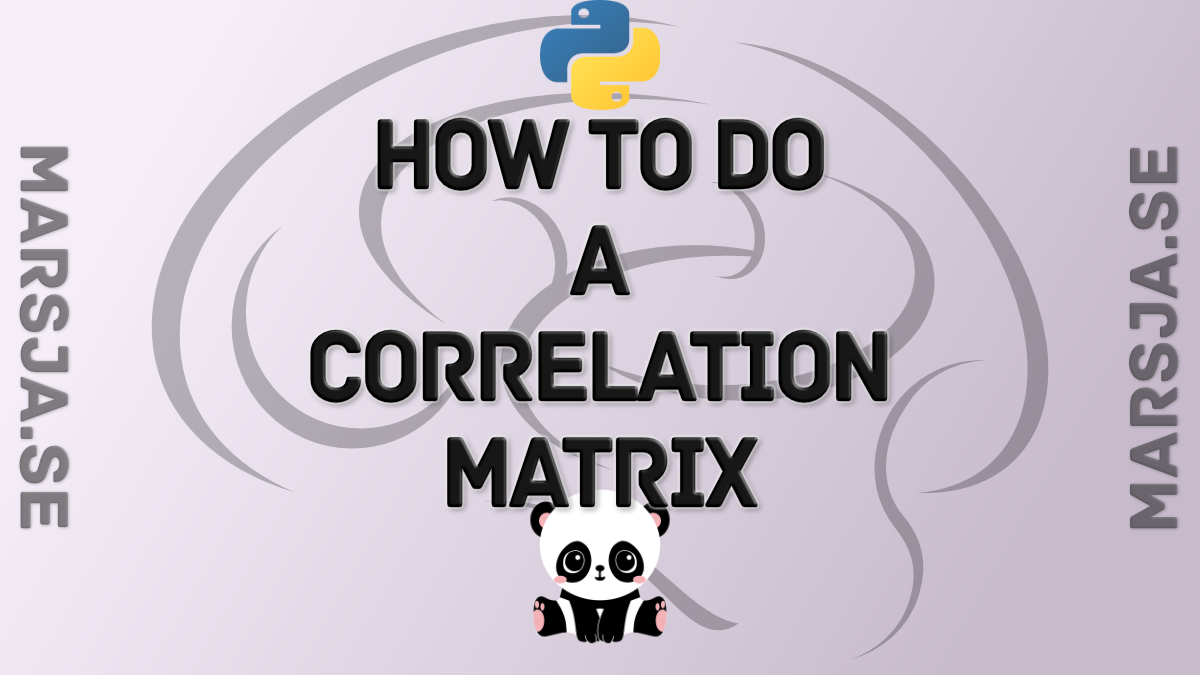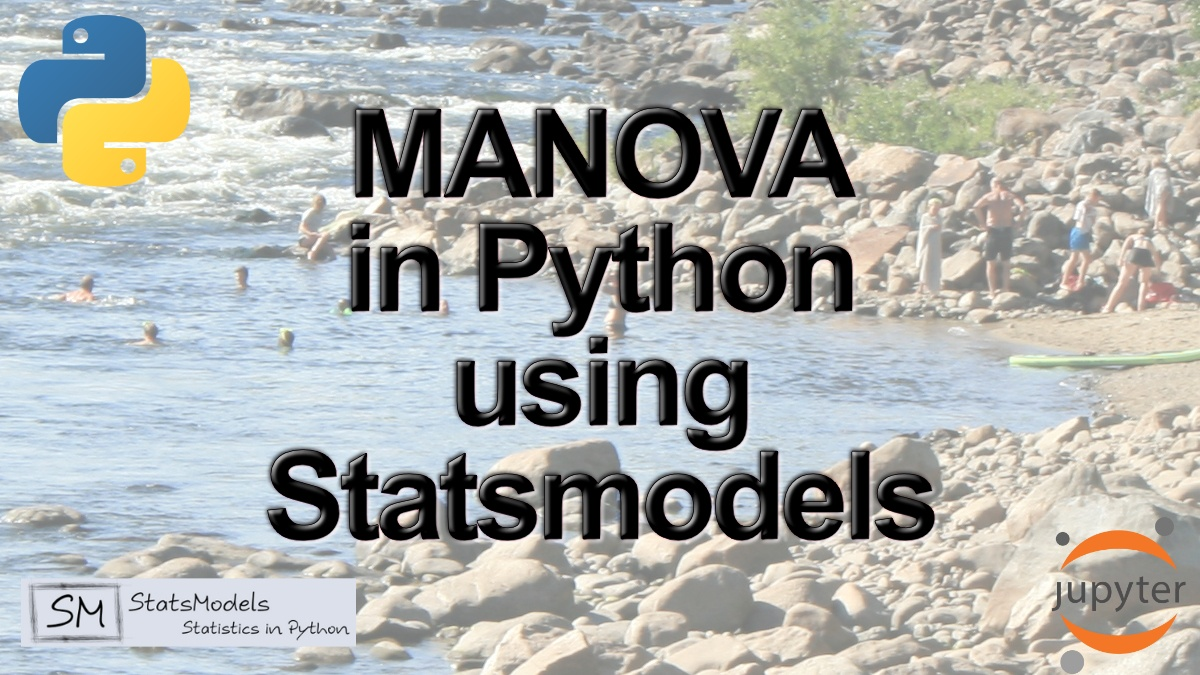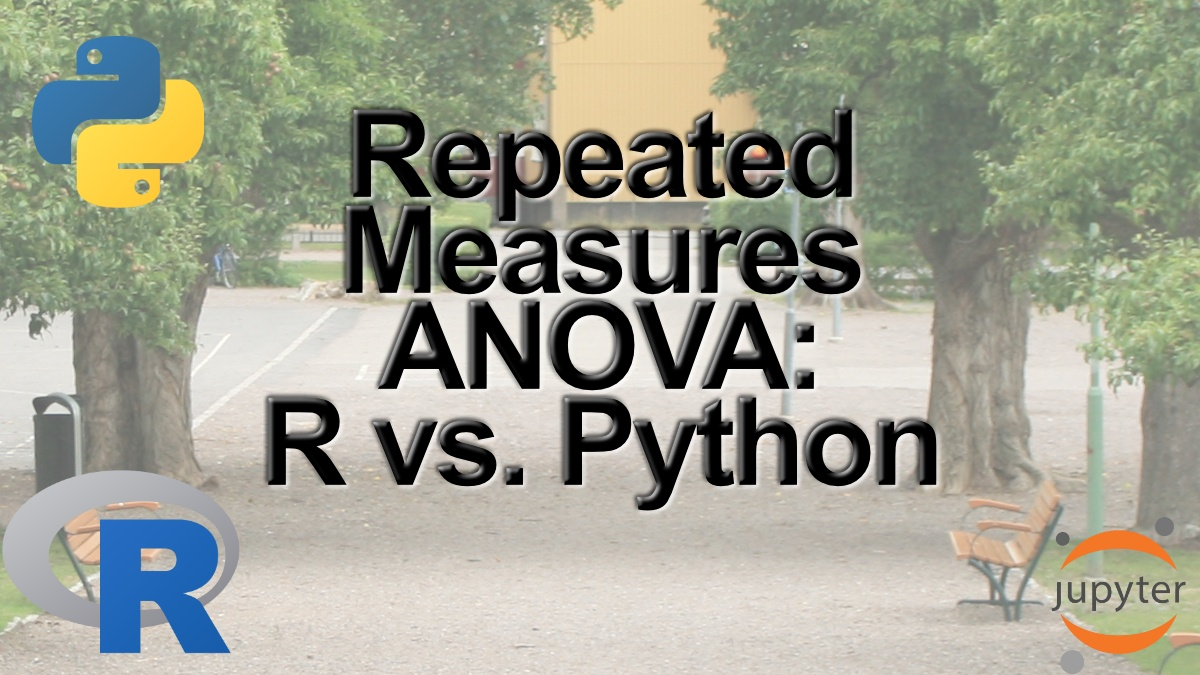## Create a Correlation Matrix in Python with NumPy and Pandas

In this post, we will go through how to calculate a correlation matrix in Python with NumPy and Pandas. Now, there will be a number of Python correlation matrix examples in this tutorial. First, we will read data from a CSV fil so we can, in a simple way, have a look...## How to use Pandas get_dummies to Create Dummy Variables in Python

In this post, we will learn how to use Pandas get_dummies() method to create dummy variables in Python. Dummy variables (or binary/indicator variables) are often used in statistical analyses as well as in more simple descriptive statistics. Towards the end of the...## Python MANOVA Made Easy using Statsmodels

In previous posts, we learned how to use Python to detect group differences on a single dependent variable. However, there may be situations in which we are interested in several dependent variables. In these situations, the simple ANOVA model is inadequate. One way...## Repeated Measures ANOVA in R and Python using afex & pingouin

In this post, we will learn how to carry out repeated measures Analysis of Variance (ANOVA) in R and Python. To be specific, we will use the R package afex and the Python package pingouin to carry out one-way and two-way ANOVA for within-subject’s design. The...

## Probabilistic Programming in Python

Learn about probabilistic programming in this guest post by Osvaldo Martin, a researcher at The National Scientific and Technical Research Council of Argentina (CONICET) and author of Bayesian Analysis with Python: Introduction to statistical modeling and...